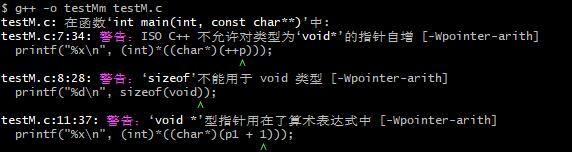（1）指针的大小
（2）指针的自加和自减
（3）指针大小和一块内存的大小

#include <iostream>

using namespace std;

int main(int argc, char const *argv[])
{
cout << sizeof(char *) << " " << sizeof(int *) << " " << sizeof(long *) << endl;
return 0;
}


32位环境下你将看到如下输出：
4 4 4

64位环境下，你将看到以下输出：
8 8 8

（1）错误认为指针的大小取决于前面类型限定名的大小。一定要谨记定义指针的类型限定名只是影响指针指向的内存单元的数据长度，与指针的大小无关怎么理解呢？看下图：

#include <iostream>

using namespace std;

int main(int argc, char const *argv[])
{

int x = 0x12345678; //小端序： 78 56 34 12
cout << hex << (int)*((char*)&x) << endl; //输出 56 由于指针强转位char*, 所以访问数据长度为一个字节，即第一个字节
cout << hex << (int)*((short*)&x) << endl; //输出 5678 由于指针强转位short*, 所以访问数据长度为两个字节，即第一和第二个字节

return 0;
}

#include <stdio.h>

int main(int argc, char const *argv[])
{

int x = 0x12345678;  //大部分常见的CPU都为小端序，包括当前测试用机：故内存中十六进制形式： 78 56 34 12
char *p = (char*)&x;
printf("0x%x\n", (int)*(++p));  //输出 0x56   ++p 一次跳了一个字节，即 sizeof(char) 个字节

short *p1 = (short*)&x;
printf("0x%x\n", (int)*((char*)(++p1)));  //输出 0x34   ++p1 一次跳了两个字节， 即 sizeof(short) 个字节

return 0;
}

#include <stdio.h>

int main(int argc, char const *argv[])
{
int x = 0x12345678;  //大部分常见的CPU都为小端序，包括当前测试用机：故内存中十六进制形式： 78 56 34 12
void *p = &x;   //变为void
printf("%x\n", (int)*((char*)(++p)));  //输出 56     ++p 一次跳了一个字节，即 sizeof(void) 个字节
printf("%d\n", sizeof(void));    //输出 1

void *p1 = &x;   //变为void
printf("%x\n", (int)*((char*)(p1 + 1)));  //输出 56    ++p1 一次跳了一个字节， 即 sizeof(void) 个字节
return 0;
}
gcc编译运行输出结果：
56
1
56（1）sizeof()不能用于void
（2）ISO C++ 不允许对类型为‘void*’的指针自增 [-Wpointer-arith]
（3）‘void *’型指针用在了算术表达式中 [-Wpointer-arith]

#include <stdio.h>
#include <stdlib.h>

/***************************************
请给出以下程序的执行结果。
***************************************/
int main(int argc, char const *argv[])
{
char *p = (char *)malloc(100);
long *p1 = (long *)malloc(100 * sizeof(long));
char arr;

printf("%d  %d  %d\n", sizeof(p), sizeof(p1), sizeof(arr));
return 0;
}

32位运行环境： 4  4  200
64位运行环境： 8  8  200

【原创内容，转载请注明出处】
It's

*转载请注明出处，严禁非法转载。
https://www.devsong.org
QQ留言 邮箱留言